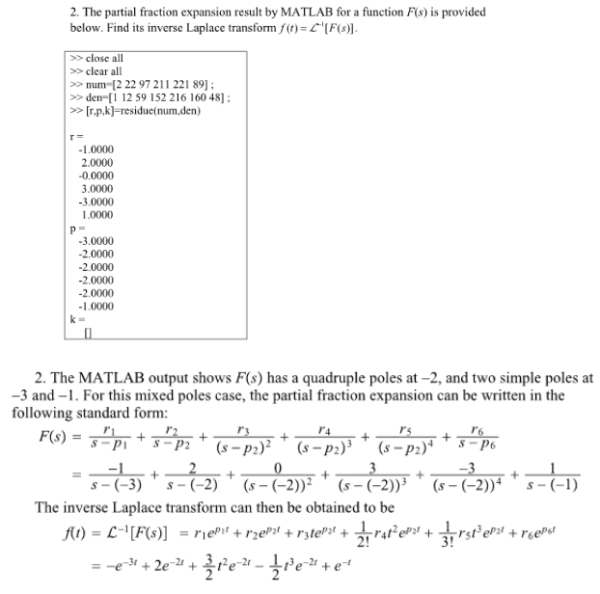The partial fraction expansion reslut by MATLAB for a function F(s) is provided below. Find its inverse Laplace transform.The partial fraction expansion reslut by MATLAB for a function F(s) is provided below. Find its inverse Laplace transform.

System Dynamics Page 2 dynamics dynamics dynamics dynamics dynamics dynamics dynamics System dynamics Page 3